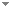Switch to: References

# Mice with finitely many Woodin cardinals from optimal determinacy hypotheses

Journal of Mathematical Logic 20 (Supp01):1950013 (2020)

 In Inner Models with Woodin Cardinals.Sandra Müller & Grigor Sargsyan - 2021 - Journal of Symbolic Logic 86 (3):871-896.We analyze the hereditarily ordinal definable sets $\operatorname {HOD}$ in $M_n[g]$ for a Turing cone of reals x, where $M_n$ is the canonical inner model with n Woodin cardinals build over x and g is generic over $M_n$ for the Lévy collapse up to its bottom inaccessible cardinal. We prove that assuming $\boldsymbol \Pi ^1_{n+2}$ -determinacy, for a Turing cone of reals x, $\operatorname {HOD} ^{M_n[g]} = M_n,$ where $\mathcal {M}_{\infty }$ is a direct limit of iterates of $M_{n+1}$, (...) Direct download (2 more)     Export citationBookmarkLong Games and Σ-Projective Sets.Juan P. Aguilera, Sandra Müller & Philipp Schlicht - 2021 - Annals of Pure and Applied Logic 172 (4):102939.We prove a number of results on the determinacy of σ-projective sets of reals, i.e., those belonging to the smallest pointclass containing the open sets and closed under complements, countable unions, and projections. We first prove the equivalence between σ-projective determinacy and the determinacy of certain classes of games of variable length <ω^2 (Theorem 2.4). We then give an elementary proof of the determinacy of σ-projective sets from optimal large-cardinal hypotheses (Theorem 4.4). Finally, we show how to generalize the proof (...) Direct download (2 more)     Export citationBookmark1 citation Projective Games on the Reals.Juan P. Aguilera & Sandra Müller - 2020 - Notre Dame Journal of Formal Logic 61 (4):573-589.Let Mn♯ denote the minimal active iterable extender model which has n Woodin cardinals and contains all reals, if it exists, in which case we denote by Mn the class-sized model obtained by iterating the topmost measure of Mn class-many times. We characterize the sets of reals which are Σ1-definable from R over Mn, under the assumption that projective games on reals are determined:1. for even n, Σ1Mn=⅁RΠn+11;2. for odd n, Σ1Mn=⅁RΣn+11.This generalizes a theorem of Martin and Steel for L, (...) Direct download (3 more)     Export citationBookmark1 citation